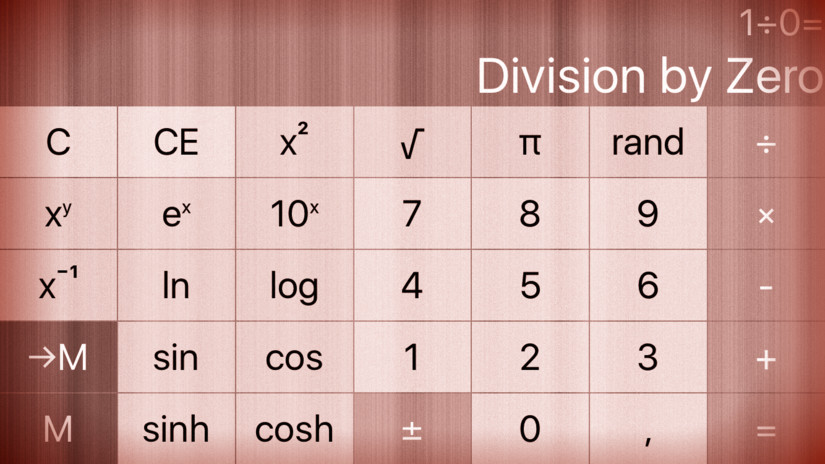# cs193p – Assignment #2 Extra Task #1Starting with the calculator brain, we could adjust the existing operations to include the error handling. Because most operations do not need error handling, create a new structure to hold the error functions:

```    private enum ErrorOperation {
case unaryOperation((Double) -> String?)
case binaryOperation((Double, Double) -> String?)
}

private let errorOperations: Dictionary<String,ErrorOperation> = [
"√": ErrorOperation.unaryOperation({ 0.0 > \$0 ? "SQRT of negative Number" : nil }),
"÷": ErrorOperation.binaryOperation({ 1e-8 >= fabs(\$0.1) ? "Division by Zero" : nil }),
"x⁻¹" : ErrorOperation.unaryOperation({ 1e-8 > fabs(\$0) ? "Division by Zero" : nil }),
"ln" : ErrorOperation.unaryOperation({ 0 > \$0 ? "LN of negative Number" : nil }),
"log" : ErrorOperation.unaryOperation({ 0 > \$0 ? "LOG of negative Number" : nil }),
"x!" : ErrorOperation.unaryOperation({ 0 > \$0 ? "Factorial of negative Number" : nil })
]
```

We will use those to set and return an error message string in the evaluate method:

```    func evaluate(using variables: Dictionary<String,Double>? = nil)
-> (result: Double?, isPending: Bool, description: String, error: String?)
{
var error: String?
...
return (result, nil != pendingBinaryOperation, description ?? "", error)
}
}
```

For operations with a single operand, we call the error message directly – if it exisits:

```                    case .unaryOperation(let function, let description):
if nil != accumulator {
if let errorOperation = errorOperations[symbol],
case .unaryOperation(let errorFunction) = errorOperation {
error = errorFunction(accumulator!.0)
}
...
}
```

For binary operations we have to wait till we get the second operand – thus we handle errors when we perform the pending binary operation. … and need to store the symbol of the current operation:

```        struct PendingBinaryOperation {
let symbol: String
...
}

func performPendingBinaryOperation() {
if nil != pendingBinaryOperation && nil != accumulator {
if let errorOperation = errorOperations[pendingBinaryOperation!.symbol],
case .binaryOperation(let errorFunction) = errorOperation {
error = errorFunction(pendingBinaryOperation!.firstOperand.0, accumulator!.0)
}
...
}
}
```

… and provide the symbol during the evaluation:

```                    case .binaryOperation(let function, let description):
...
pendingBinaryOperation = PendingBinaryOperation(symbol: symbol, function: function, description: description, firstOperand: accumulator!)
...
```

This way the view controller still works without changing anything.

We want to know the errors, therefore display them when they occur:

```    private func displayResult() {
...
if let error = evaluated.error {
display.text = error
} else if let result = evaluated.result {
displayValue = result
}
...
}
```

The complete code for the assignment #2 extra task #1 is available on GitHub.